Email Us: info@lupinepublishers.comCall Us: +1 (914) 407-6109   57 West 57th Street, 3rd floor, New York - NY 10019, USA

# Lupine Publishers Group

## Lupine Publishers

Submit Manuscript

ISSN: 2690-5779# Existence and Uniqueness of the solution of large deflection of circular plate by the Burger’s method Volume 1 - Issue 3

Doo Sung Lee*

• Department of Mathematics College of Education, Konkuk University, Seoul, Korea

Received: October 28, 2020   Published: January 29, 2021

Corresponding author: Doo-Sung Lee, Department of Mathematics College of Education, Konkuk University Seoul, Korea

## Abstract

The existence and uniqueness of the solutions to an infinite system of nonlinear equations arising in the dynamic analysis of large deflection of a circular plate are discussed. Under the condition that the rim of the plate is prevented from inplane motions, explicit equation for the coupling parameter is given.

Keywords: Existence; uniqueness; Berger’s method;1991 Mathematics Subject Classification; 46(Functional analyss);73(Mechanics of solids)

## Introduction

In the analysis of classical mechanics problems, there are cases where linear mathematical model can not fully describe the phenomena. If the deflection of the plate is of order of magnitude of its thickness, the differential equations for the deflection and displacements can be written in terms of nonlinear equations. These nonlinear equations are usually difficult to obtain the solution. Thus, several attempts have been tried to obviate the difficulties.
Among these attempts, it was Berger’s method which drew much attention. Berger derived as implified nonlinear equations for a plate with large deflections by assuming that the strain energy due to the second invariants of the middle surface strains can be neglected when deriving the differential equations by energy method. Berger restricted his analysis to static and isotropic cases.
Later, his procedure was generalized to dynamics of isotropic plates by Nash and Modeer  and to dynamic phenomena in anisotropic plates and shallow shells by Nowinski . Berger’methods is dealt in recent books  and . In the research paper, Banerjee and Mazumdar review various approximate methods including Berger’s in relation to the investigation of geometrically nonlinear problems. In Sathymoorthy and Chia , a nonlinear vibration theory is formulated for rectilinearly orthotropic circular plates using Berger’s method. On the other hand Han and Petyt report that the large vibration of in-plane membrane forces over the plate span for some of the laminated plates has been observed which will definitely affect the application of Berger’s hypothesis to the geometrically nonlinear analysis of these laminated plates.
There are many other papers giving explicit solutions to various cases, however the search for the existence and uniqueness of the solution is rare, thus it is the purpose of this paper to discuss this matter. We now briefly go over the Berger’s method for the circular plate. The deformation of the middle surface pertinent to the large transverse deflections is described by the equations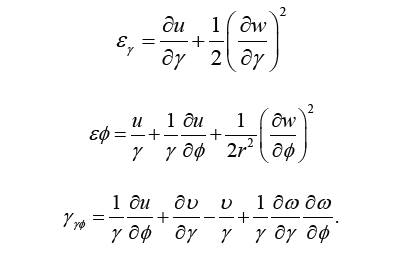In the above equations w = deflection of plate in the normal direction. u, v =displacement in plane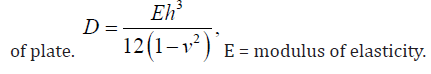The strain energy due to the bending can be written aswhere S denotes the surface of the circular plate. We can write the strain energy due to the stretching of the middle plane as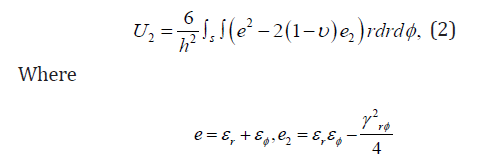The variation of the work by the external force is now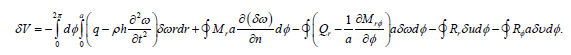By virtue of d’Alembert’s principle the motion of the structure is replaced by a state of static equilibrium governed by by the equation of minimum potential energy of the system,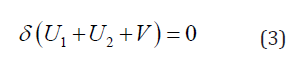Burger’s method is to set e2 = 0 in (2) as it is relatively negligible compared to other terms, and equation (3) then gives following equations,from which integration of (4) gives the interesting result the first strain invariant is a constant. The
governing equations are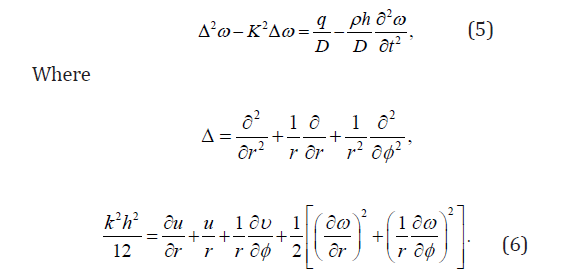We consider a clamped circular plate so that boundary conditions are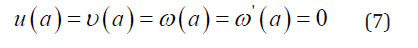The coupling parameter is now determined from the equation (6) and boundary conditions (7)
as followsWe attempt to find Fourier series solutions to (5) when q = 0.Here ( ), ( ) n n J γ I γ are the Bessel functions of the first kind and modified Bessel function of the first kind, respectively. When this solution is substituted in (5) and (8) we findWe discuss the existence and uniqueness of the solutions to above infinite system of nonlinear equations. The initial conditions on (11) will be taken as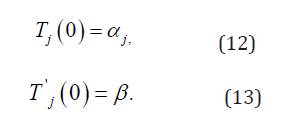If we multiply (11) by T0jand sum j from 0 to infinity, we can show that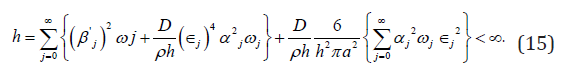At first glance it would appear that if the initial conditions (12) and (13) satisfy a finite energy condition, i.e.,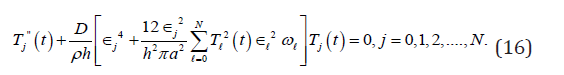then (11) should have a solution for all t > 0. Indeed this is the case for finite system of the form (11) since the finite systemhas associated with it a Lipschitz constant. Therefore, successive approximation method may be applied to prove the existence of solution to (16). However, the infinite system of equations (11) is not Lipschitz continuous since the coefficients of Tj is unbounded as j →∞. Thus the method of successive approximation fails and an alternative procedure is necessary.

In section 2, it will be shown that under the initial conditions (12) and (13) solution of the finite system (16) converge to a solution (11) as N → ∞. In section 3 it will be shown that the solution of (11) satisfying initial conditions (12) and (13) is unique.

## Existence

To prove solution existence of (11), we define a set of functions Tj>, N in the following way: for j ≤ N, Tj>, N is a solution of the finite system of equations (16) satisfying the initial conditions (12) and (13) for j = 0, 1, 2, · · ·, N and for j > N set Tj>, N = 0. The functions Tj>, N are solutions of the infinite system (11),i.e.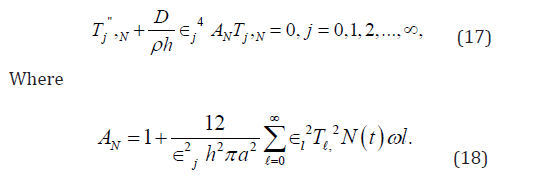If in addition the initial data (12) and (13) satisfy the finite energy condition (15) it follows That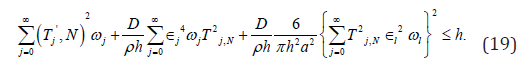Thus there exist constants M1, M2 and M3 independent of NLemma 1. |A0N | is uniformly bounded independent of N where prime indicates differentiation with respect to t. Proof. After differentiating the function AN, if we employ Schwarz inequality we obtain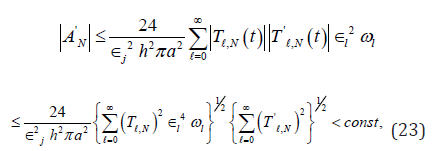in view of the relations (20) and (21).
Lemma 2. |AN | is uniformly bounded independent of N
Proof. |AN | is uniformly bounded independent of N from the relation (22).
Thus AN is uniformly bounded and equicontinuous; by Arzela’s lemma, there exists a subsequence {ANi} which converges uniformly to a continuous function A(t). Let Tj be the solution of the(linear) equation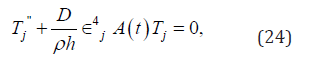satisfying the initial conditions (12) and (13). The existence of solutions to (11) is settled by the following theorem.

Theorem 1. The infinite system of (11) have a solution satisfying the initial data (12) and (13). Proof. It is only necessary to show that the solutions of linear system (24) furnish a solution of system (11). For this purpose it suffices to show that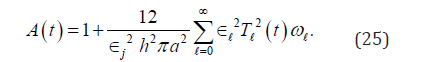The series which occurs in (25) converges since(cf.(22))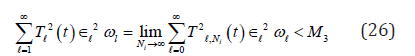The equality in (25) follows from the estimate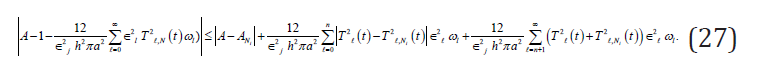The right side of (27) can be made arbitrarily small by first choosing n, then choosing Ni.

## Uniqueness

In this section it will be shown that the infinite system (11) has at most one solution satisfying the initial conditions (12) and (13). We write (11) in the following way.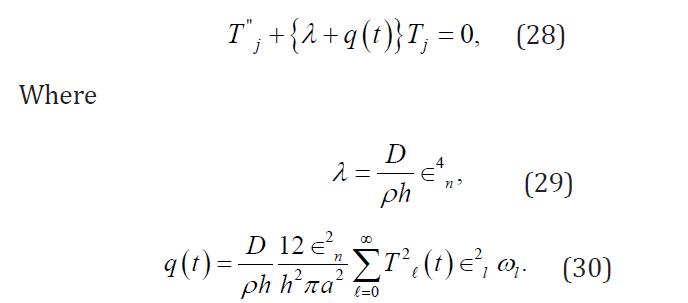Let Tj and Sj be solutions of (11) satisfying the initial conditions (12) and (13) i.e. Tj is the solution of (28) with q(t) being given by (30) and Sj is the solution of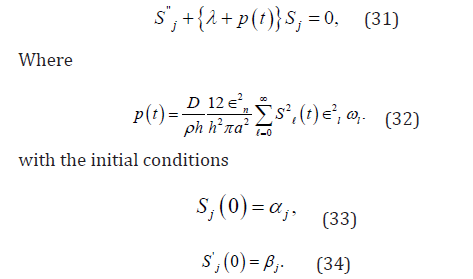If we multiply (28) by Sj and from the resulting equation, we substract(31) multiplied by Tj and integrate it from zero to infinity, we get, after integrating by parts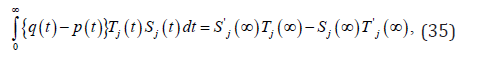where we have used the initial conditions (12),(13),(33),(34). According to Gel’fand and Levitan there exists function K(t, x) having continuous partial derivatives of first and second order such that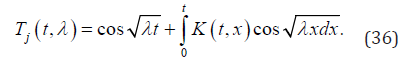If we substitute (36) into (28),we find that the partial differential equation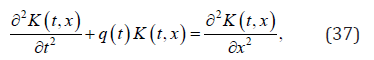and the boundary conditions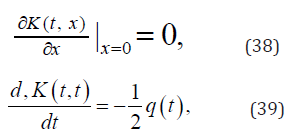are satisfied. Similarly,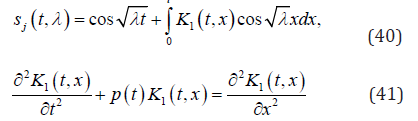and the boundary conditionsThus if we substitute (36) and (40) into (35), we find that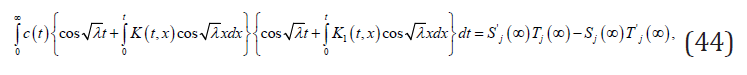where c(t) = q(t) − p(t). Making change of variables and changing the order of integration in (44) we get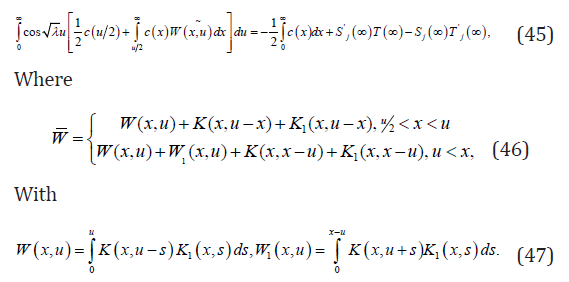The left hand side of (45) is a function of λ,whereas right hand side is a constant. The equality holds only when both sides are equal to zero. Thus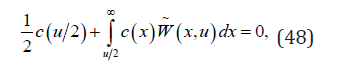and from Gronwall’s Lemma we get c(t) = 0, i.e.,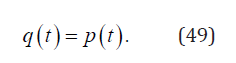Let Uj = Kj − Sj . We show Uj = 0 in the following. From (28) and (31) we havebecause of (49). Let us denoteThen Vj (t) will be the solution of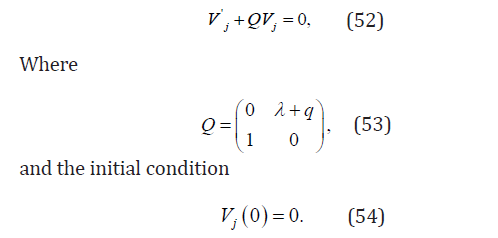With the initial condition (54), the solution of (52) is Vj = 0 from the semi-group theory. So we have the following theorem.
Theorem 2.The system of equations (11) have at most one solution satisfying the initial conditions (12) and (13).

1. HM Berger, A new approach to the analysis of large deflections of plates, JAppl.Mech. 22(1955): 465-472.
2. WANash, JR Modeer(1960) Certain approximate analyses of non-linear behavior of plates and shallow shells. ProcSymp ThThin ElShells, Interscience, New York, USA.
3. JLNowinski(1974)Some static and dynamic problems concerning non-linear behavior of plates and shallow shells with discontinuous boundary conditions. IntJNon-Linear Mechanics 10: 1-14.
4. ARoy and RKBera(2019) Linear and nonlinear deformations of elastic solids, CRC press. Taylor & Francis Group.
5. FBloom, DCoffin(2000) Handbook of Thin Plate Buckling and Postbuckling, Chapman & Hall/CRC New York.
6. MMBanerjee, J Mazudar(2016)A review of methods for linear and nonlinear vibration analysisof plates and shells, Procedia Engineering 144: 493-503.
7. MSathymoorthy, CY Chia (1982) Nonlinear flexural vibration of moderately thick orthotropic circular plates. Ingenieur-Archiv 52: 237-243.
8. WHan, MPetyt (1997) Geometrically nonlinear vibration analysis of thin, rectangular plates using the hierarchical finite element method-II:!st mode of laminated plates and higher modes of isotropic and laminated plates, Computers and Structures. 63: 309-318.
9. IM Gel’fand and BM Levitan (1955) On the determination of a differential equation from its spectral function. 21: 253-304.
Close## Online Submission System

### Submit ManuscriptClick here for Manuscript Sample Templates### Drag and drop files here

or

( For multiple files submission, zip them in a single file to submit. For file zipping software Download )